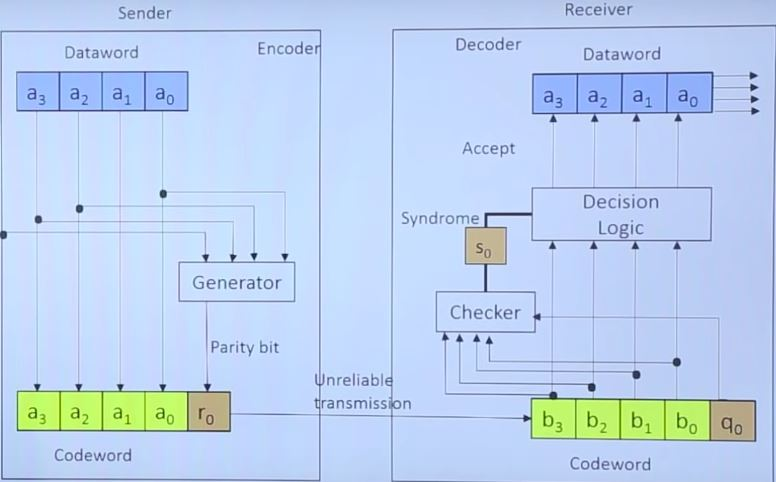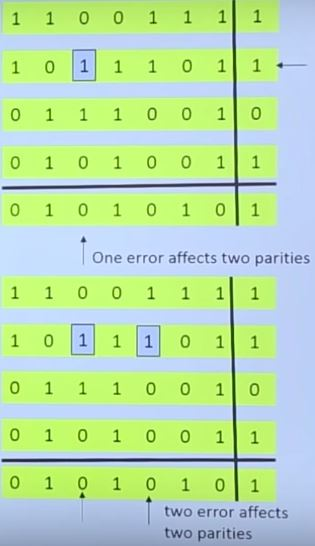## Recent Post

Errors in data transmission in one or multiple bits are unavoidable. To detect or correct errors, we need to send extra (redundant) bits with data.

Let us consider following probabilities -

Pb : Probability that a bit is received in error; also known as the bit error rate (BER)

P1 : Probability that a frame arrives with no bit errors

P2 : Probability that, with an error-detecting algorithm in use, a frame arrives with one or more undetected errors

P3 : Probability that, with an error-detecting algorithm in use, a frame arrives with one or more detected bit errors but no undetected bit errors

When there is no detection algorithm then P3 = 0 And

P1 = (1 - Pb)f
P2 = (1 - P1)

f is the number of bits per frame

# Encoder & decoder for simple parity-check code -# Single and double bits errors in two-dimensional parity check code -• # The checksum is sent with the data

• The message (including checksum) is divided into 16-bit words.
• The sum is complemented and becomes the new checksum.
• If the value of checksum is 0, the message is accepted; otherwise, it is rejected.

#### 1 comment:

1.According to Stanford Medical, It is really the ONLY reason this country's women live 10 years longer and weigh 19 KG lighter than we do.

(By the way, it is not about genetics or some secret diet and really, EVERYTHING to do with "how" they eat.)

BTW, What I said is "HOW", not "WHAT"...